# Home Work Set # 4, Physics 217, Due: October 3, 2001

### Problem 1

Find the electrostatic potential inside and outside a uniformly charged solid sphere whose radius is R and whose total charge is q. Use infinity as your reference point. Compare the gradient of V in each region, and check that it yields the correct electric field.

### Problem 2

Find the electrostatic potential a distance r from an infinitely long straight wire that carries a uniform line charge λ. Compute the gradient of your potential, and check that it yields the correct electric field (calculated using Gauss's law).

### Problem 3

A conical surface (an empty ice-cream cone) carries a uniform surface charge σ. The height of the cone is a, as is the radius of the bottom (see Figure 1). Find the potential difference between points P (the vertex) and Q (the center of the bottom).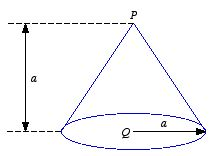Figure 1. Problem 3.

### Problem 4

Find the potential on the axis of a uniformly charged solid cylinder, a distance z from the center. The length of the cylinder is L, its radius is R, and the charge density is ρ. Use your result to calculate the electric field at this point. (Assume that z > L/2.)

### Problem 5

a) Three charges are situated at the corners of a square (side s) as shown in Figure 2. How much work does it take to bring in another charge, + q, from far away and place it at the fourth corner?
b) How much work does it take to assemble the whole configuration of these four charges?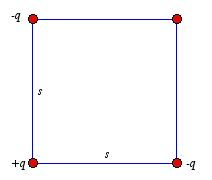Figure 2. Problem 5.

### Problem 6

Find the energy stored in a uniformly charged solid sphere of radius R and total charge q. Do it in three different ways:
a) Use the following equation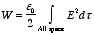and the electric field generated by a uniformly charged sphere.
b) Use the following equation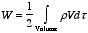and the electrostatic potential of a uniformly charged sphere.
c) Use the following equation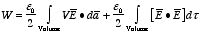and take as integration volume a sphere of radius a. What happens as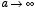?

### Problem 7

In this problem we will explore a fourth way of calculating the electrostatic potential energy of the uniformly charged sphere of problem 6. Assemble the sphere layer by layer, each time bringing in an infinitesimal charge dq from far away and smearing it uniformly over the surface, thereby increasing the radius. How much work dW does it take to build up the radius by an amount dr? Integrate this to find the work necessary to put together the entire sphere of radius R and total charge q. Compare your answer with the results of Problem 6.